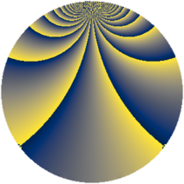# Properties

 Label 931.2.xLevel $931$ Weight $2$ Character orbit 931.x Rep. character $\chi_{931}(226,\cdot)$ Character field $\Q(\zeta_{9})$ Dimension $378$ Newform subspaces $9$ Sturm bound $186$ Trace bound $5$

# Related objects

## Defining parameters

 Level: $$N$$ $$=$$ $$931 = 7^{2} \cdot 19$$ Weight: $$k$$ $$=$$ $$2$$ Character orbit: $$[\chi]$$ $$=$$ 931.x (of order $$9$$ and degree $$6$$) Character conductor: $$\operatorname{cond}(\chi)$$ $$=$$ $$133$$ Character field: $$\Q(\zeta_{9})$$ Newform subspaces: $$9$$ Sturm bound: $$186$$ Trace bound: $$5$$ Distinguishing $$T_p$$: $$2$$, $$3$$

## Dimensions

The following table gives the dimensions of various subspaces of $$M_{2}(931, [\chi])$$.

Total New Old
Modular forms 606 426 180
Cusp forms 510 378 132
Eisenstein series 96 48 48

## Trace form

 $$378 q + 3 q^{2} + 3 q^{3} + 3 q^{4} + 3 q^{5} + 12 q^{6} - 12 q^{8} + 3 q^{9} + O(q^{10})$$ $$378 q + 3 q^{2} + 3 q^{3} + 3 q^{4} + 3 q^{5} + 12 q^{6} - 12 q^{8} + 3 q^{9} - 15 q^{10} + 54 q^{12} + 12 q^{13} - 39 q^{15} - 9 q^{16} + 30 q^{18} - 6 q^{19} - 36 q^{22} + 3 q^{23} + 36 q^{24} + 3 q^{25} + 18 q^{26} - 30 q^{27} - 48 q^{29} - 3 q^{30} - 39 q^{31} - 6 q^{32} + 39 q^{33} + 24 q^{34} - 87 q^{36} - 6 q^{37} + 72 q^{38} + 24 q^{39} - 57 q^{40} + 30 q^{41} - 6 q^{43} - 96 q^{44} + 3 q^{45} - 12 q^{46} + 21 q^{47} + 21 q^{48} - 45 q^{50} + 3 q^{51} + 9 q^{52} - 3 q^{53} - 93 q^{54} + 27 q^{55} - 54 q^{57} + 78 q^{58} - 6 q^{59} - 228 q^{60} - 18 q^{61} + 27 q^{62} - 138 q^{64} - 21 q^{65} - 15 q^{66} + 84 q^{67} + 15 q^{68} - 42 q^{69} - 90 q^{71} - 18 q^{72} + 78 q^{73} - 3 q^{74} - 57 q^{75} - 42 q^{76} + 3 q^{78} + 15 q^{79} + 3 q^{80} - 60 q^{81} - 117 q^{82} + 18 q^{83} - 24 q^{85} + 54 q^{86} - 18 q^{87} + 45 q^{88} + 3 q^{89} + 48 q^{90} - 78 q^{92} + 39 q^{93} - 72 q^{94} - 117 q^{95} - 69 q^{96} - 3 q^{97} + 168 q^{99} + O(q^{100})$$

## Decomposition of $$S_{2}^{\mathrm{new}}(931, [\chi])$$ into newform subspaces

Label Dim $A$ Field CM Traces $q$-expansion
$a_{2}$ $a_{3}$ $a_{5}$ $a_{7}$
931.2.x.a $6$ $7.434$ $$\Q(\zeta_{18})$$ None $$3$$ $$-3$$ $$3$$ $$0$$ $$q+(\zeta_{18}+\zeta_{18}^{2}+\zeta_{18}^{3}-\zeta_{18}^{5})q^{2}+\cdots$$
931.2.x.b $6$ $7.434$ $$\Q(\zeta_{18})$$ None $$3$$ $$3$$ $$-3$$ $$0$$ $$q+(\zeta_{18}+\zeta_{18}^{2}+\zeta_{18}^{3}-\zeta_{18}^{5})q^{2}+\cdots$$
931.2.x.c $30$ $7.434$ None $$0$$ $$-6$$ $$-6$$ $$0$$
931.2.x.d $30$ $7.434$ None $$0$$ $$-6$$ $$6$$ $$0$$
931.2.x.e $30$ $7.434$ None $$0$$ $$6$$ $$-6$$ $$0$$
931.2.x.f $30$ $7.434$ None $$0$$ $$6$$ $$6$$ $$0$$
931.2.x.g $60$ $7.434$ None $$0$$ $$0$$ $$0$$ $$0$$
931.2.x.h $66$ $7.434$ None $$-3$$ $$3$$ $$3$$ $$0$$
931.2.x.i $120$ $7.434$ None $$0$$ $$0$$ $$0$$ $$0$$

## Decomposition of $$S_{2}^{\mathrm{old}}(931, [\chi])$$ into lower level spaces

$$S_{2}^{\mathrm{old}}(931, [\chi]) \cong$$ $$S_{2}^{\mathrm{new}}(133, [\chi])$$$$^{\oplus 2}$$